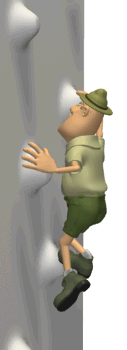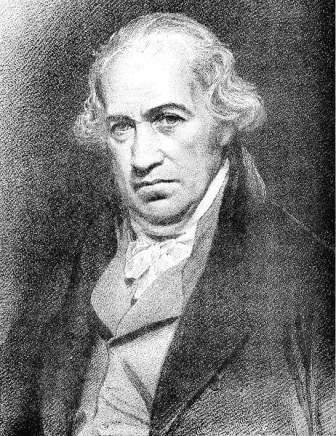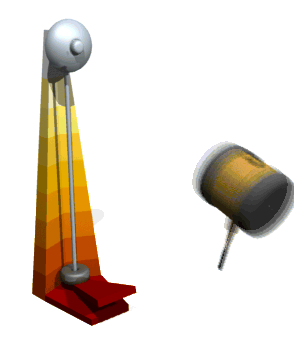HomeTry this at home!

Water that won't boil!

Turning which gear would give youWilliam Dwight Whitney The Century Dictionary and Cyclopedia: An encyclopedic Lexicon of the English Language. (New York: The Century Co., 1889)4499

Small Gear

C) Power - rate of doing work or expending energyJames Watt -
1736 - 1819

'... radically improved the power, efficiency, and cost-effectiveness of steam engines'. 'He developed the concept of horsepower, and the SI unit of power, the watt, was named after him.'  - Wikipedia

 P = W/t = Fd/t = Fv (watt) = (J/s) (N)m/s

ScalarEx 1) A force of 40 N is exerted on a mass, causing it to move with a constant speed of 5 m/s. What power is used?Ex 1) A force of 40 N is exerted on a mass, causing it to move with a constant speed of 5 m/s. What power is used?P = W/t = Fd/t = Fv (watt) = (J/s) (N)m/s

= Fv = (40 N)(5 m/s)

200 Nm/s

or 200 wattsEx 2) A girl weighing 500. newtons takes 50. seconds to climb a flight of stairs 18 meters high. Her power output vertically isEx 2) A girl weighing 500. newtons takes 50. seconds to climb a flight of stairs 18 meters high. Her power output vertically is

 F = 500. N t = 50. secs d = 18 m P = ?P = W/t = Fd/t = Fv (watt) = (J/s) (N)m/s

P = Fd/t

P = 500. N(18. m)/50. sec

P = 180 Nm/sec = 180 wattsEx 3)  A 95-kg student climbs 4.0 m up a rope in 3.0 seconds. What is the power output of the student?Ex 3)  A 95-kg student climbs 4.0 m up a rope in 3.0 seconds. What is the power output of the student?

 m = 95 kg d = 4.0 m t = 3.0 sec P = ?P = W/t = Fd/t = Fv (watt) = (J/s) (N)m/s

P = Fd/t

First find F (weight)

F = mg = 95 kg(9.81 m/s2)

= 931 N

P = [931 N(4.0 m)]/(3.0 sec)

P = 1.2 x 103 W

(Rounded to 2 significant digits)Conservation of Energy